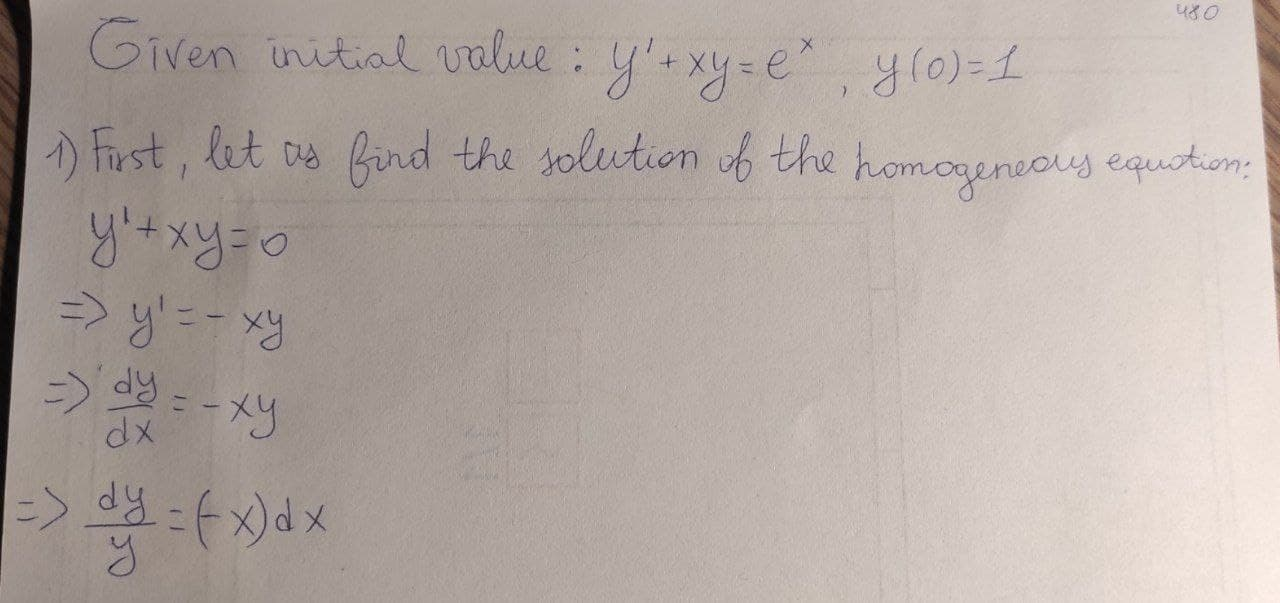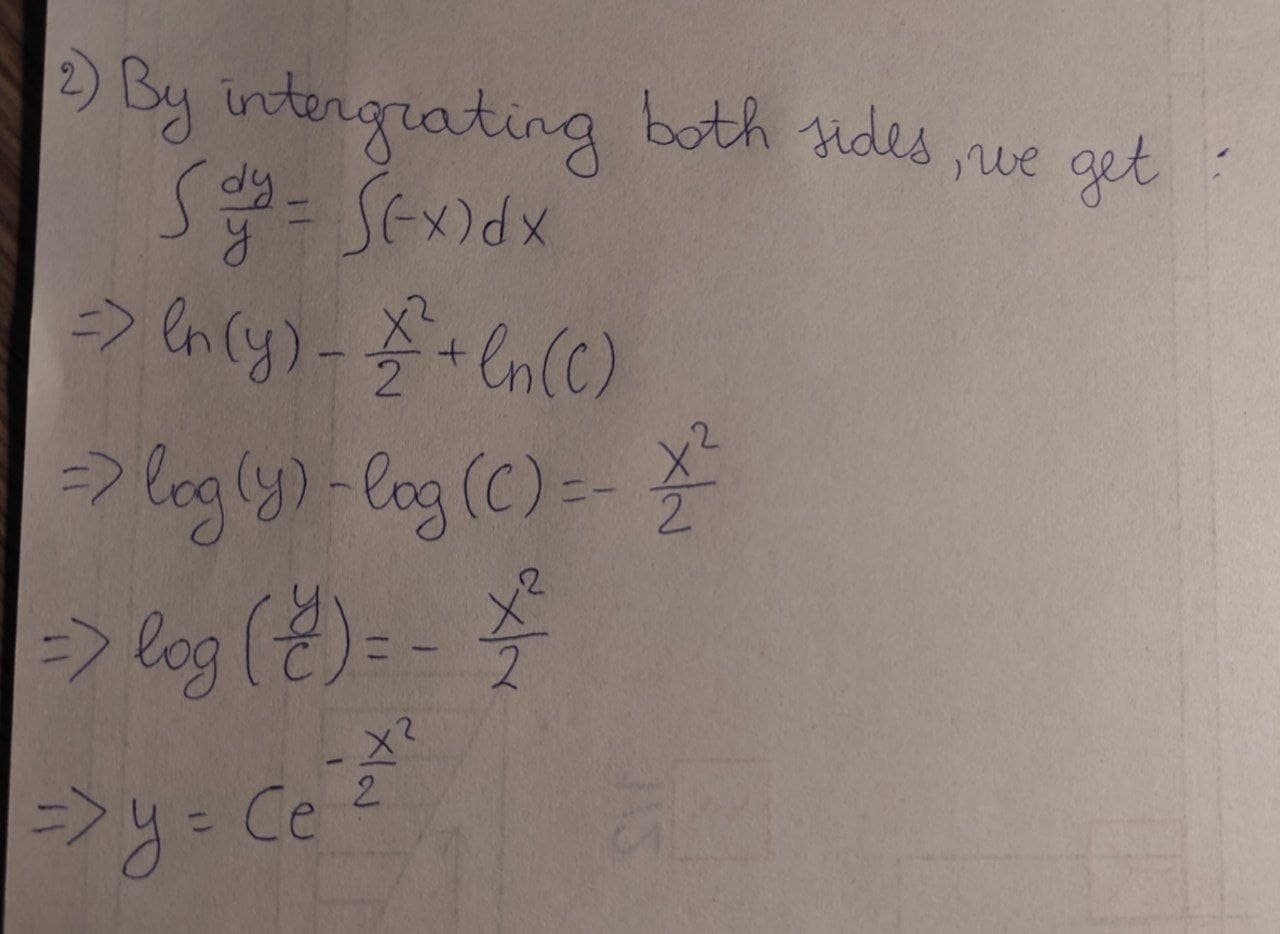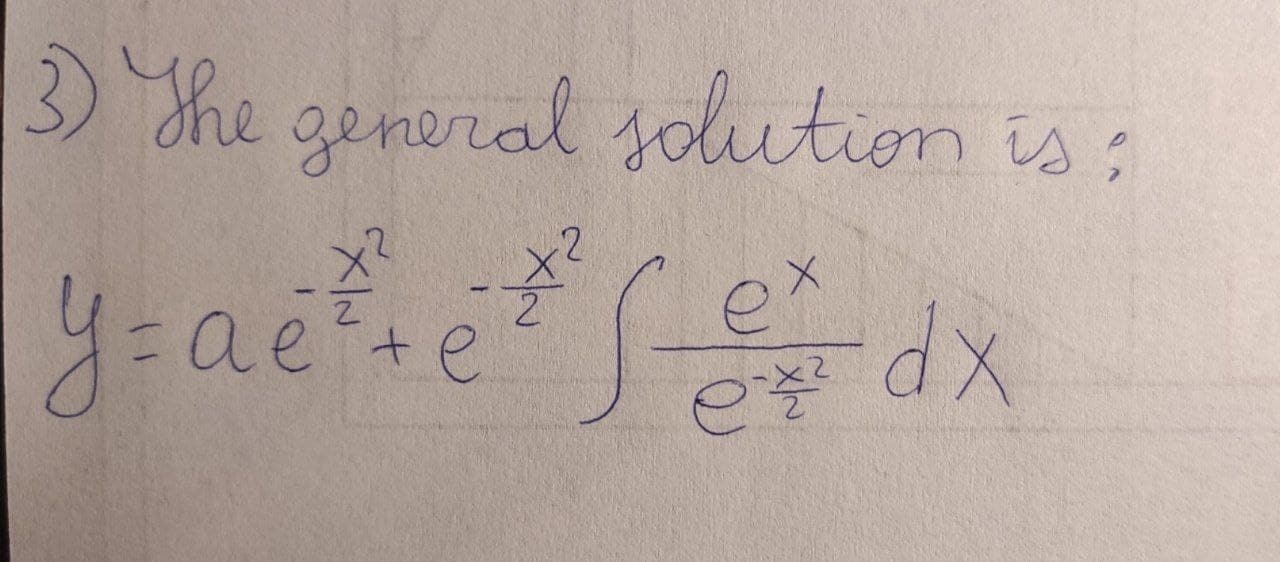# Solve this equation pls y'+xy=e^x y(0)=1Kye 2021-01-02 Answered
Solve this equation pls${y}^{\prime }+xy={e}^{x}$
$y\left(0\right)=1$
You can still ask an expert for help

• Questions are typically answered in as fast as 30 minutes

Solve your problem for the price of one coffee

• Math expert for every subject
• Pay only if we can solve itSchepperJ

First, let us find the solution of the homogeneous equation
${y}^{\prime }+xy=0$
We get,
${y}^{\prime }+xy=0$
$⇒{y}^{\prime }=-xy$
$⇒\frac{dy}{dx}=-xy$
$⇒\frac{dy}{y}=\left(-x\right)dx$
By integrating both sides, we get,
$\int \frac{dy}{y}=\int \left(-x\right)dx$
$⇒\mathrm{ln}\left(y\right)-\frac{{x}^{2}}{2}+\mathrm{ln}\left(C\right)$
$⇒\mathrm{log}\left(y\right)-\mathrm{log}\left(C\right)=-\frac{{x}^{2}}{2}$
$⇒\left(\frac{y}{C}\right)=-\frac{{x}^{2}}{2}$
$⇒y=C{e}^{\frac{{x}^{2}}{2}}$
So, the general solution of the given differential equation is given by
$a{e}^{-\frac{{x}^{2}}{2}}+{e}^{-\frac{{x}^{2}}{2}}\int \frac{{e}^{x}}{{e}^{-\frac{{x}^{2}}{2}}}dx$

###### Not exactly what you’re looking for?Jeffrey Jordon

Explanation on photo